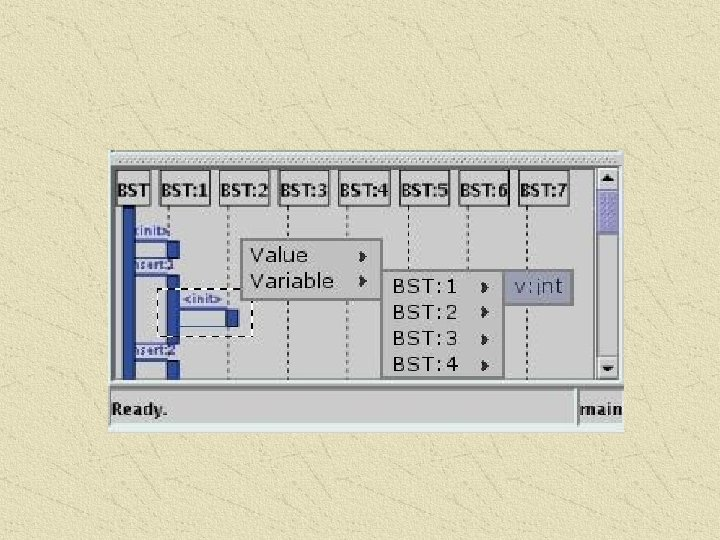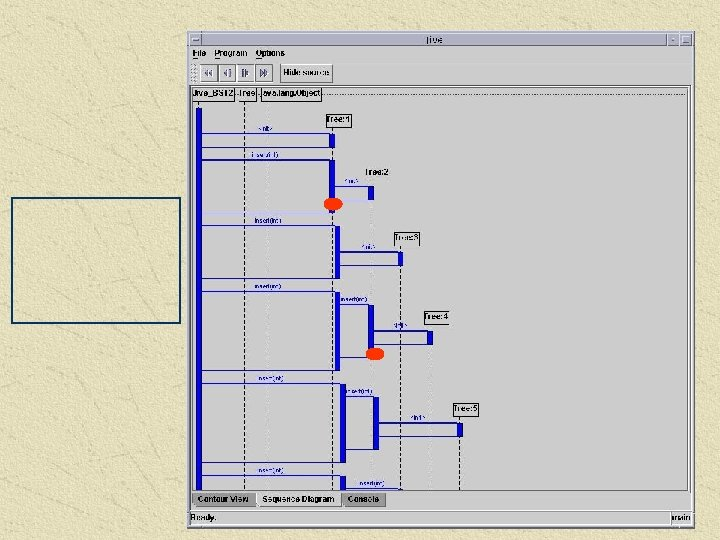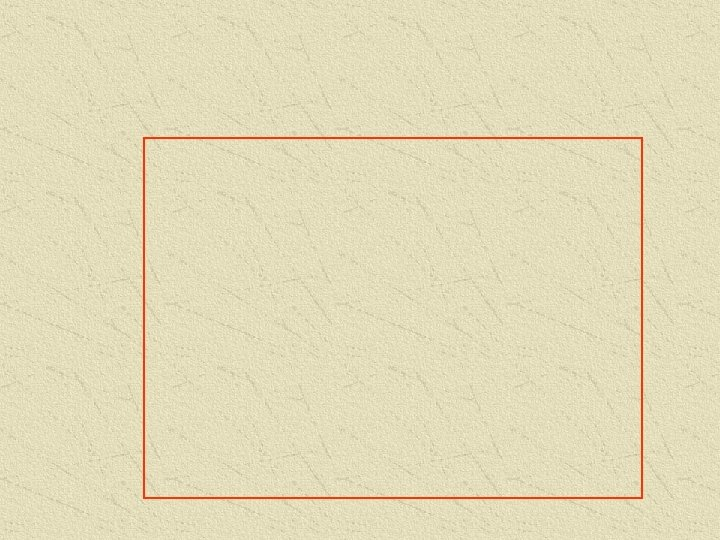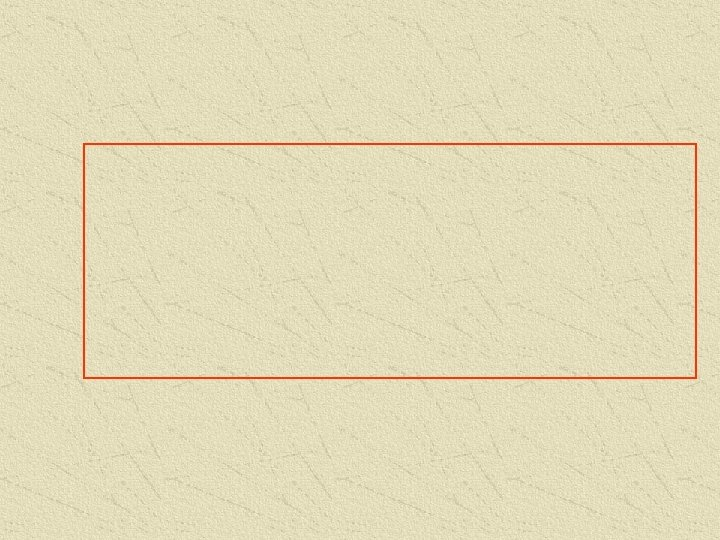Скачать презентацию Joint work with Pallavi Tambay and Paul Gestwicki

• Количество слайдов: 65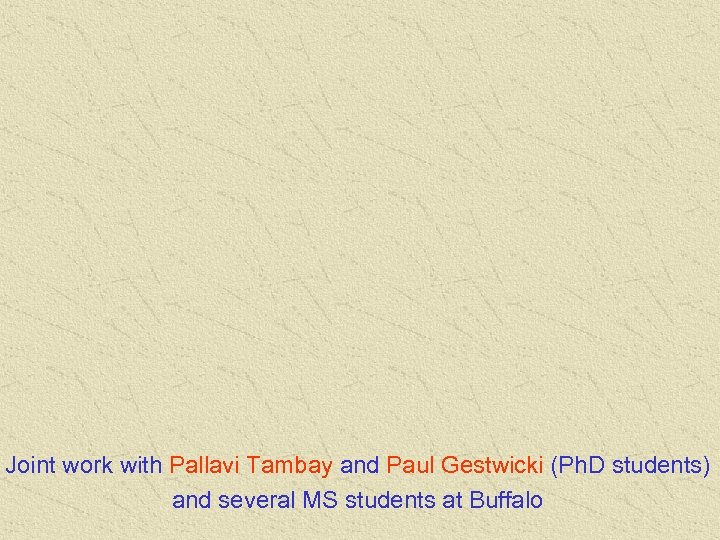Joint work with Pallavi Tambay and Paul Gestwicki (Ph. D students) and several MS students at Buffalo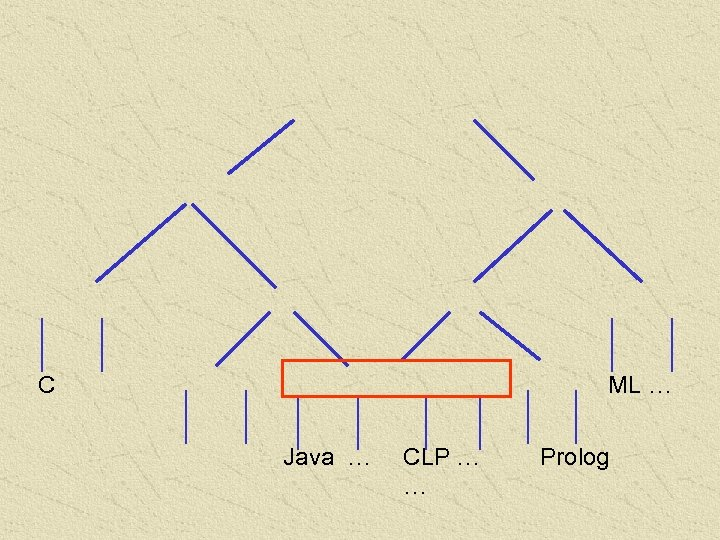C ML … Java … CLP … … Prolog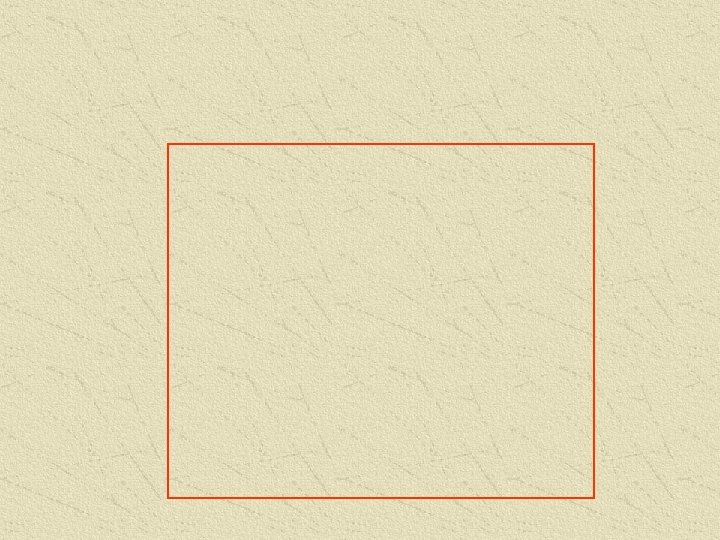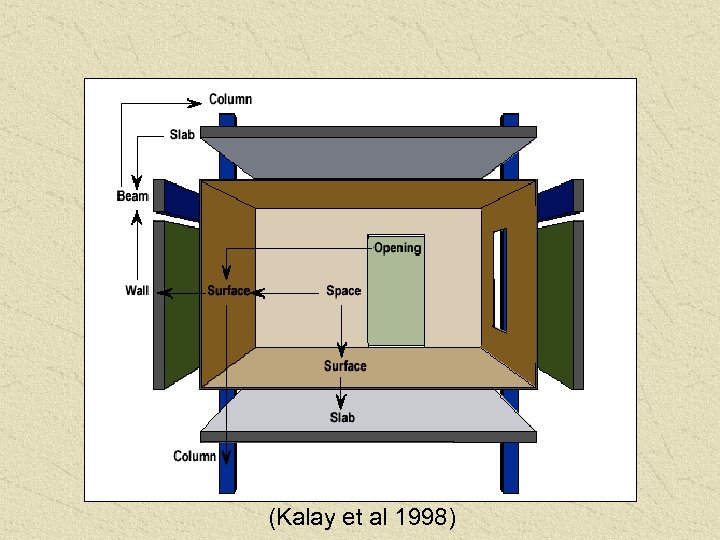(Kalay et al 1998)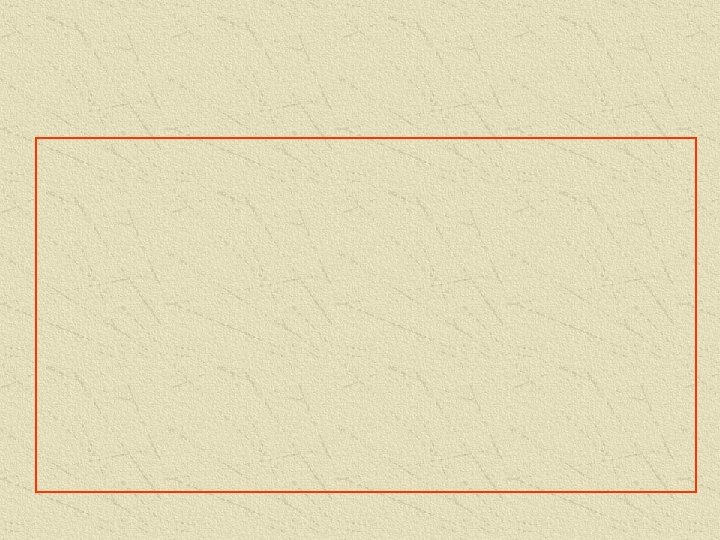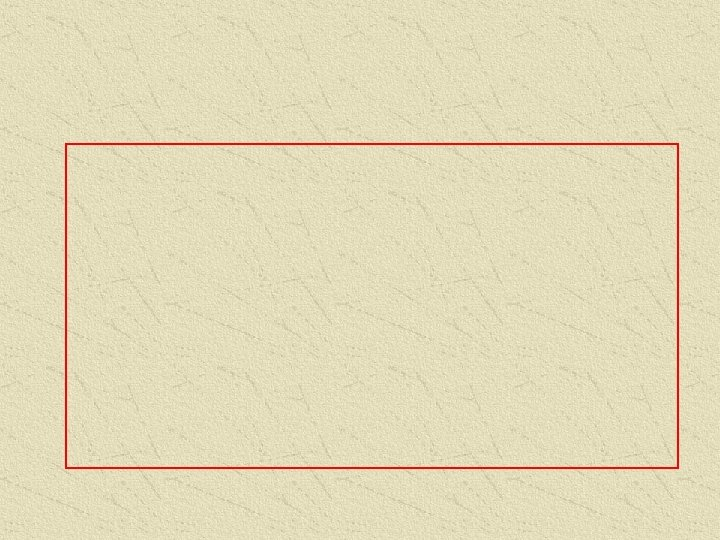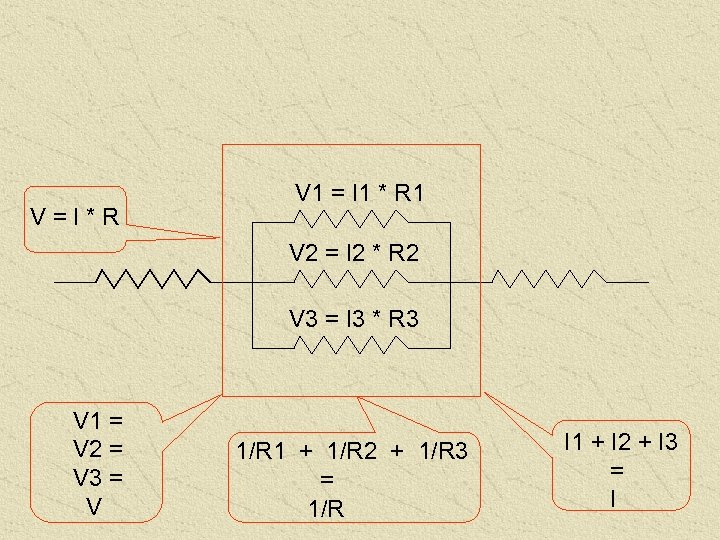V=I*R V 1 = I 1 * R 1 V 2 = I 2 * R 2 V 3 = I 3 * R 3 V 1 = V 2 = V 3 = V 1/R 1 + 1/R 2 + 1/R 3 = 1/R I 1 + I 2 + I 3 = I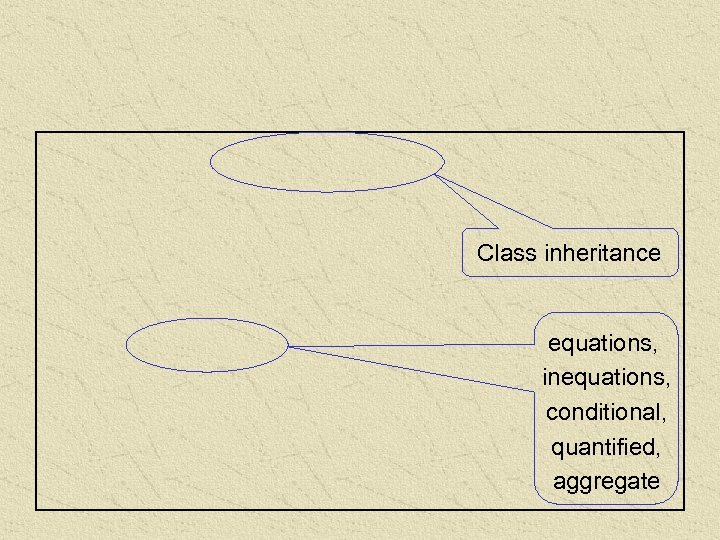Class inheritance equations, inequations, conditional, quantified, aggregate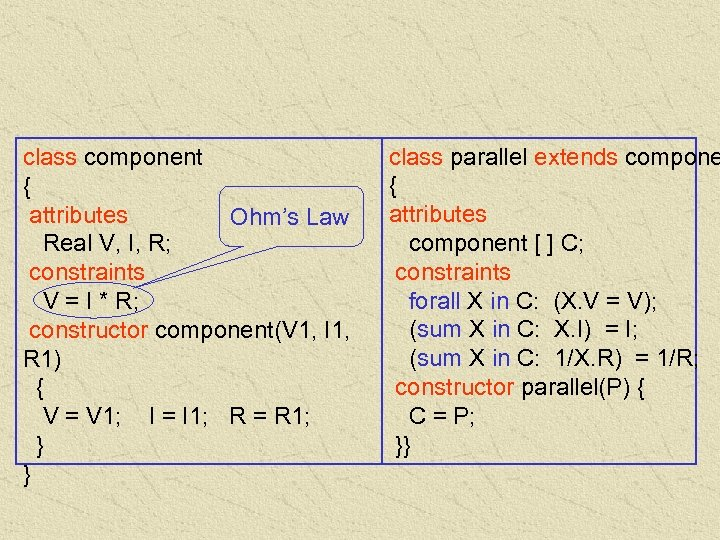class component { attributes Ohm’s Law Real V, I, R; constraints V = I * R; constructor component(V 1, I 1, R 1) { V = V 1; I = I 1; R = R 1; } } class parallel extends compone { attributes component [ ] C; constraints forall X in C: (X. V = V); (sum X in C: X. I) = I; (sum X in C: 1/X. R) = 1/R; constructor parallel(P) { C = P; }}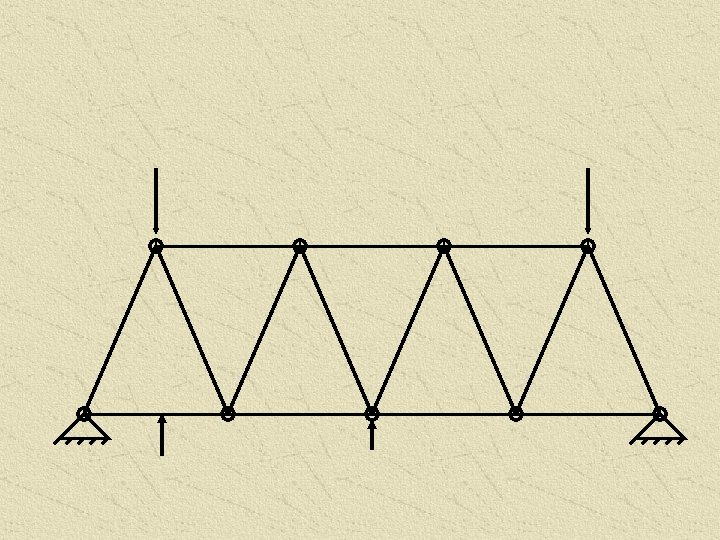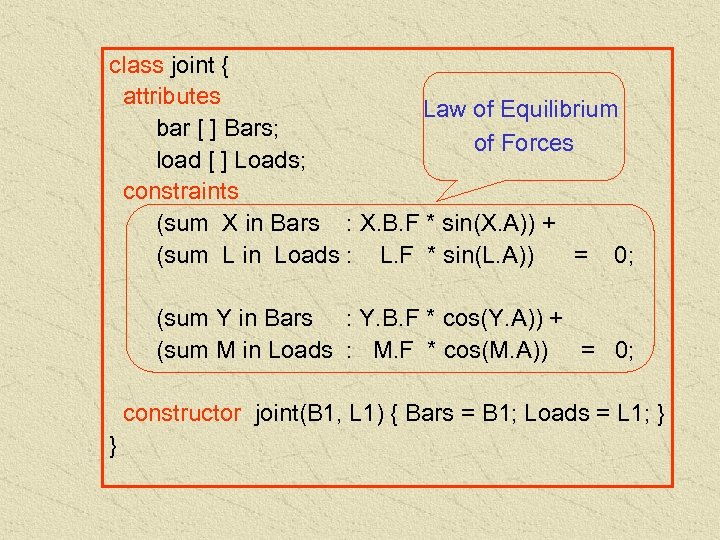class joint { attributes Law of Equilibrium bar [ ] Bars; of Forces load [ ] Loads; constraints (sum X in Bars : X. B. F * sin(X. A)) + (sum L in Loads : L. F * sin(L. A)) = 0; (sum Y in Bars : Y. B. F * cos(Y. A)) + (sum M in Loads : M. F * cos(M. A)) = 0; constructor joint(B 1, L 1) { Bars = B 1; Loads = L 1; } }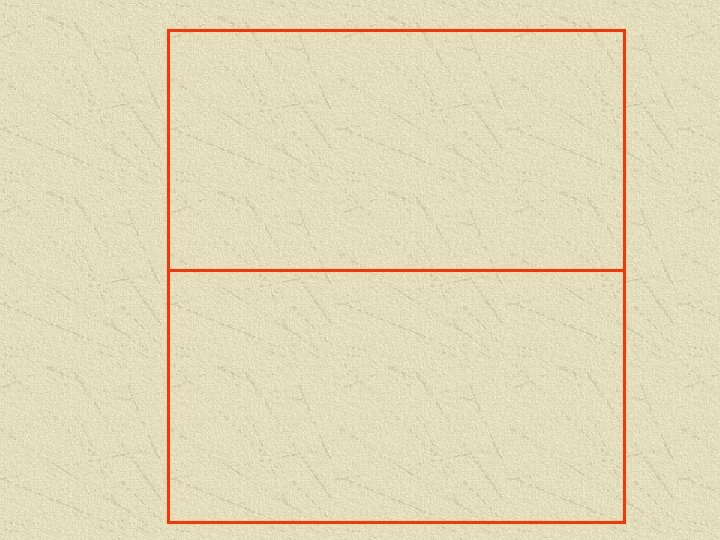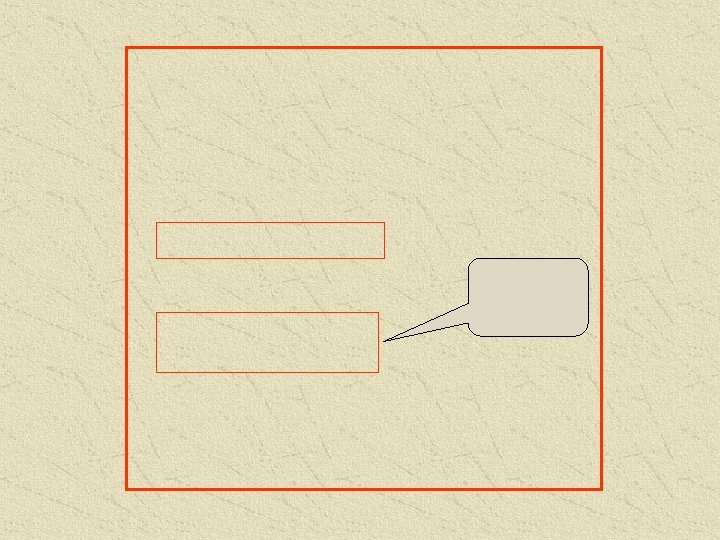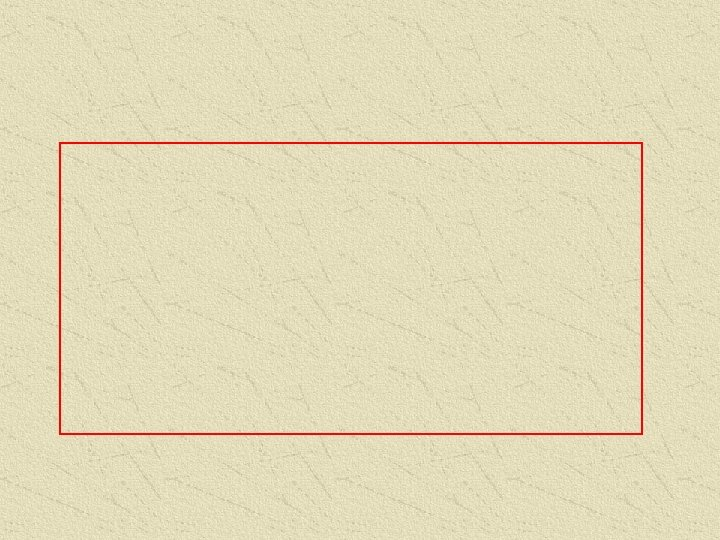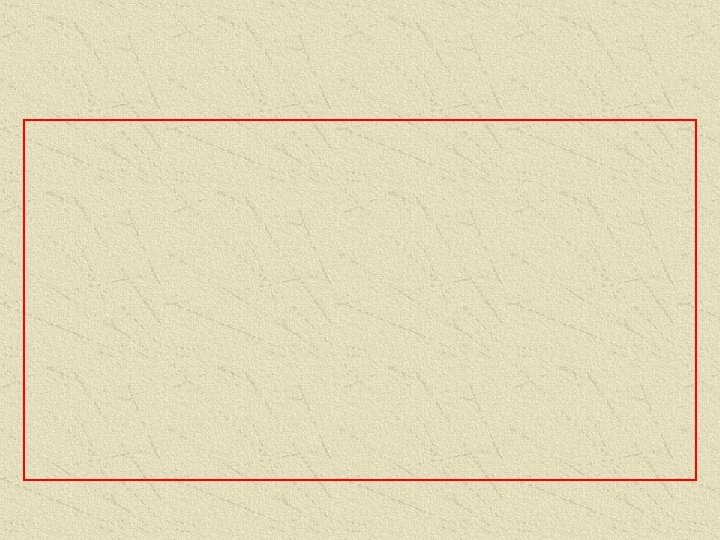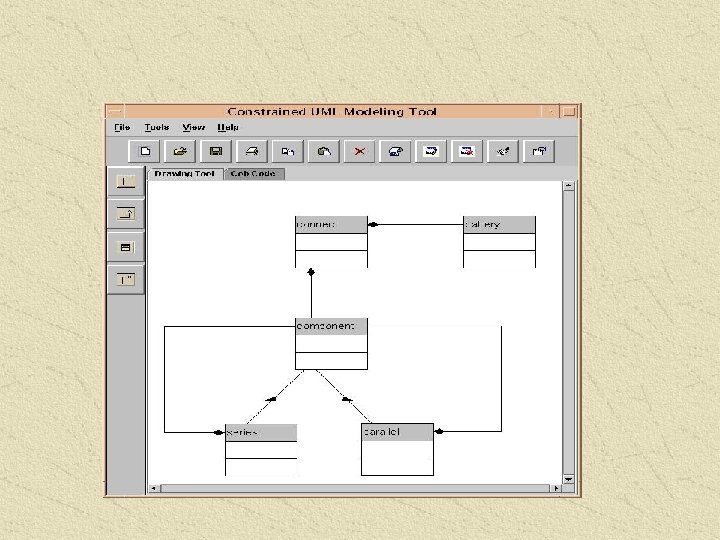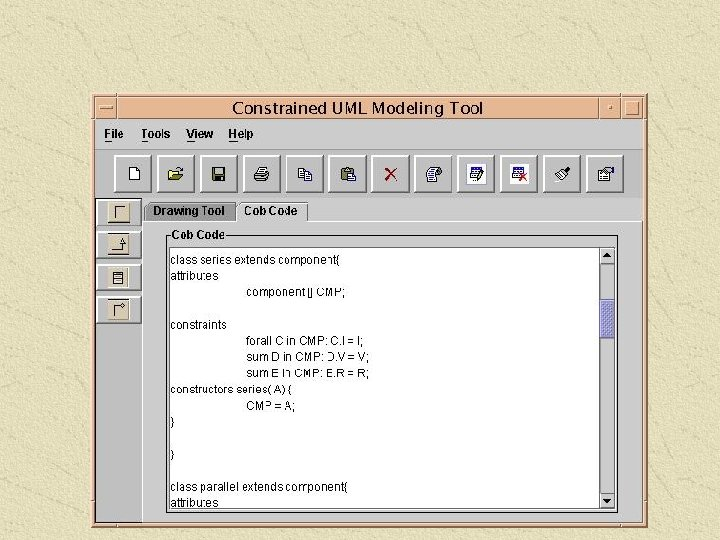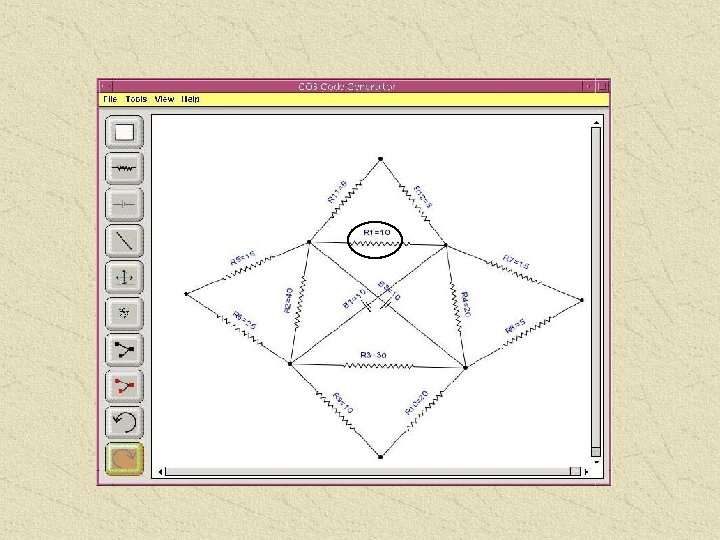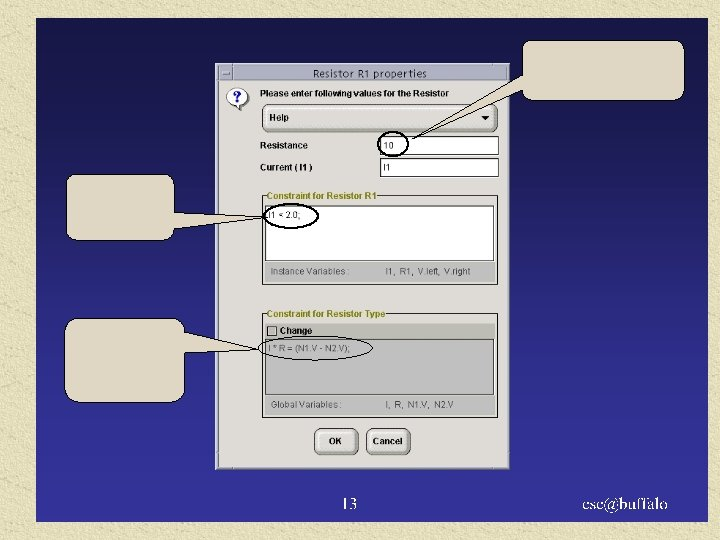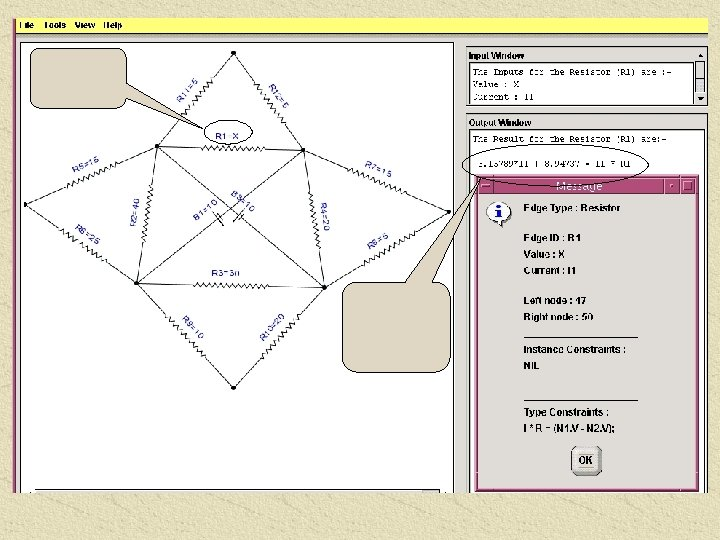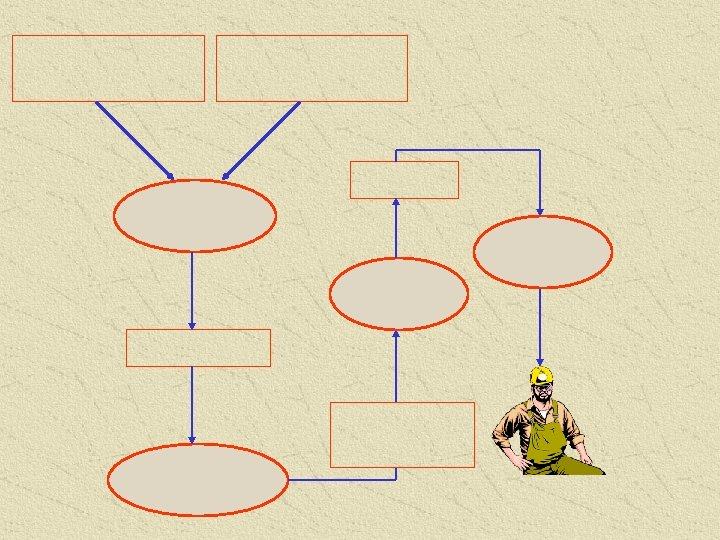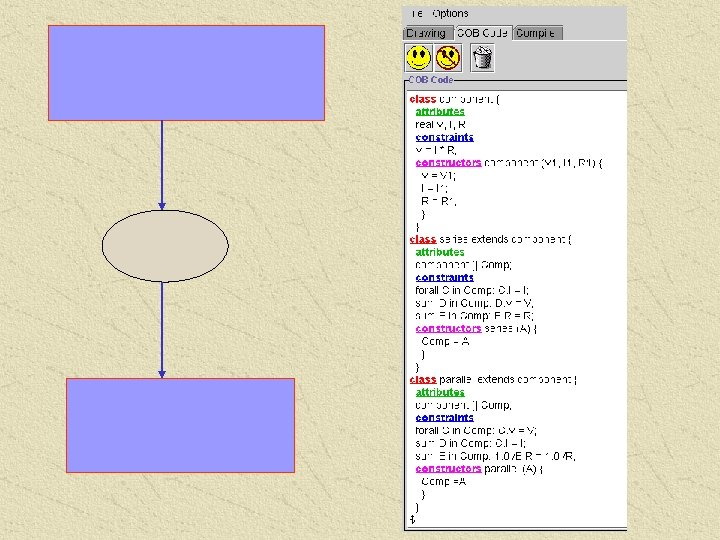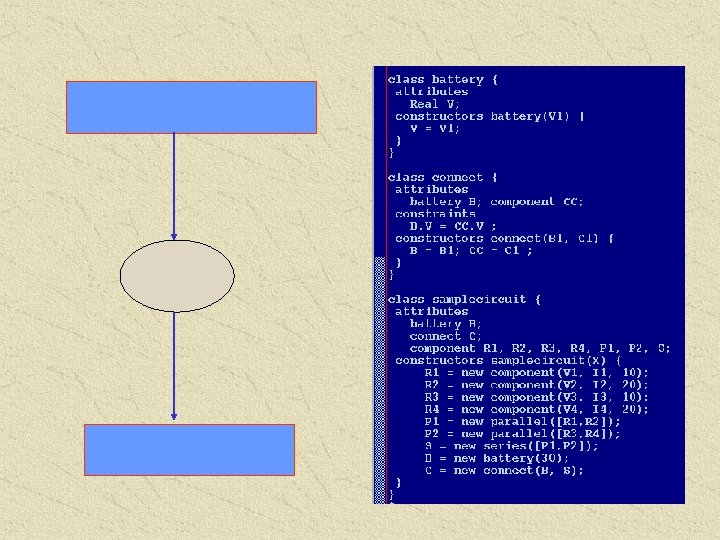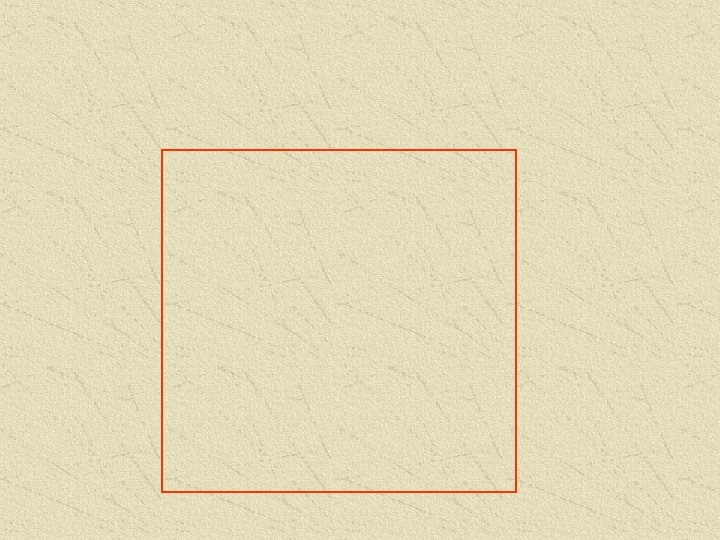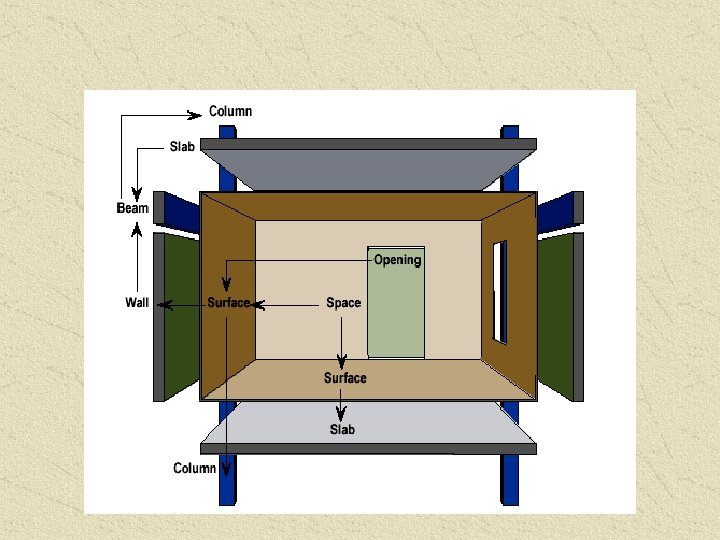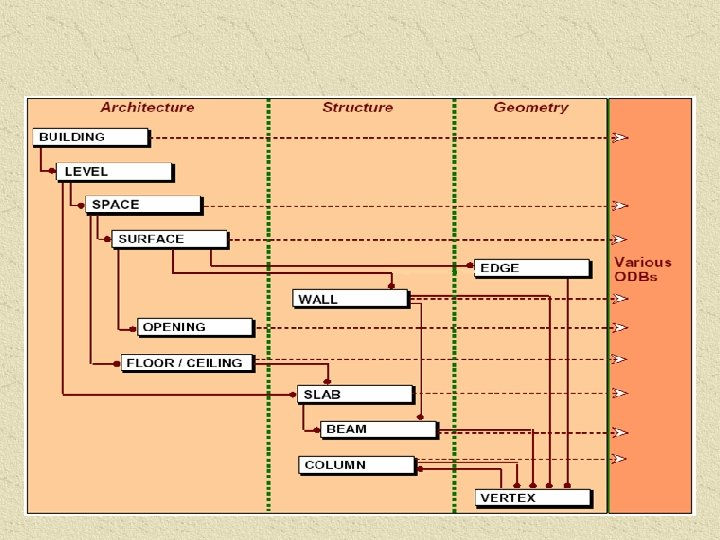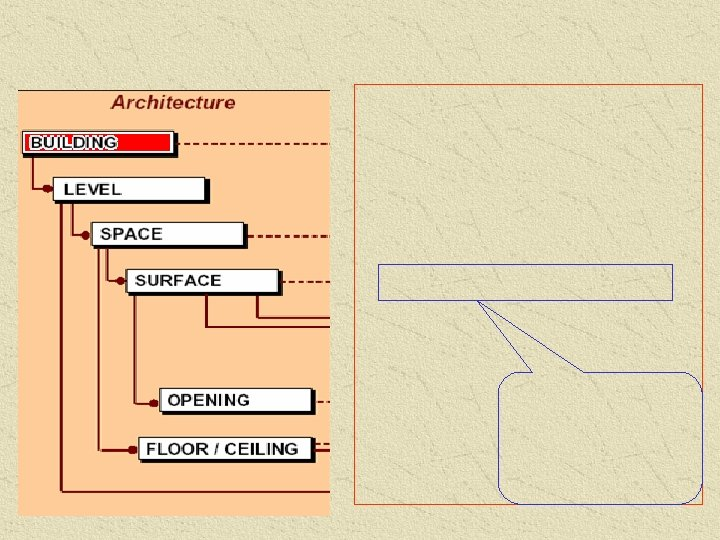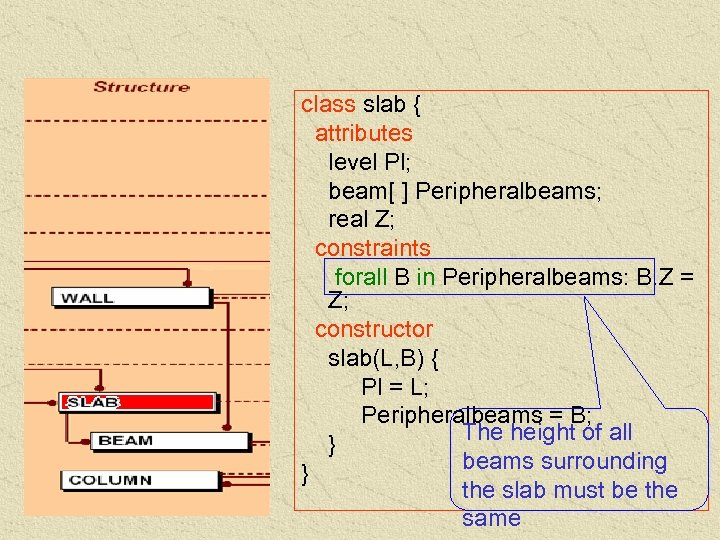class slab { attributes level Pl; beam[ ] Peripheralbeams; real Z; constraints forall B in Peripheralbeams: B. Z = Z; constructor slab(L, B) { Pl = L; Peripheralbeams = B; The height of all } beams surrounding } the slab must be the same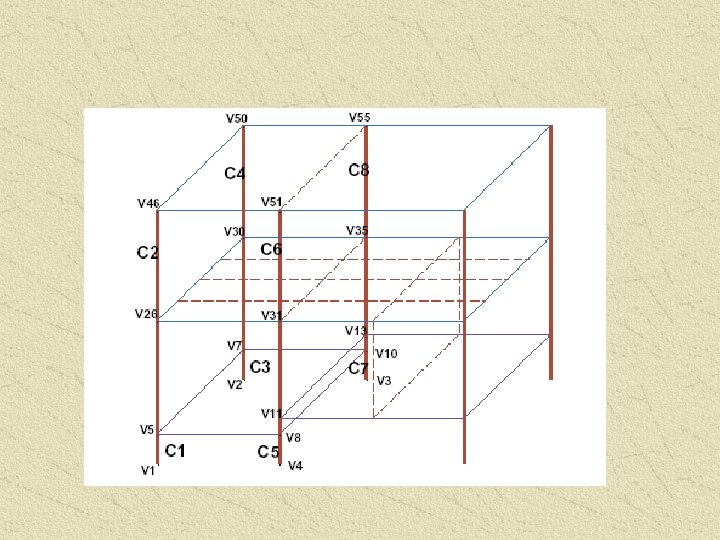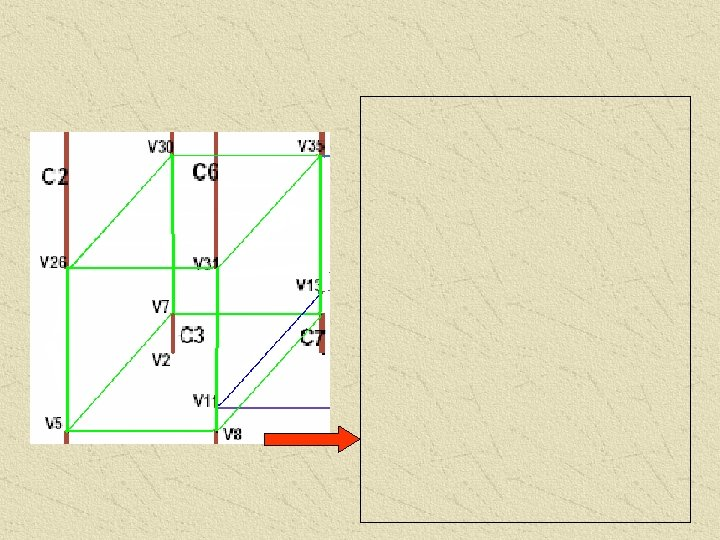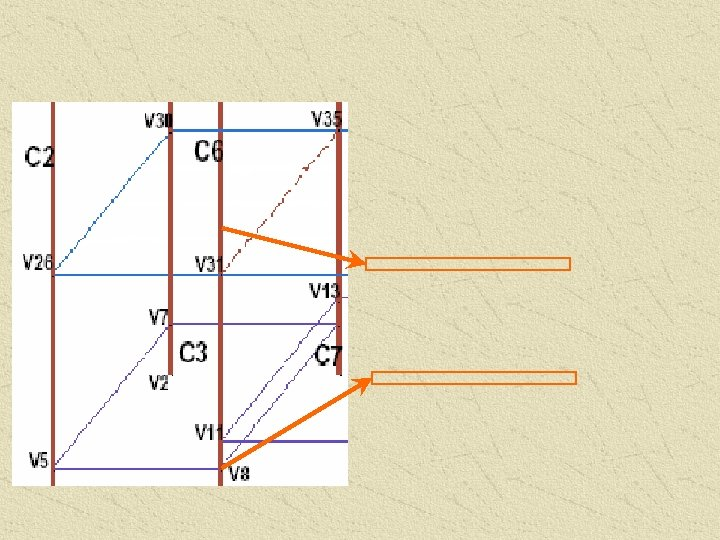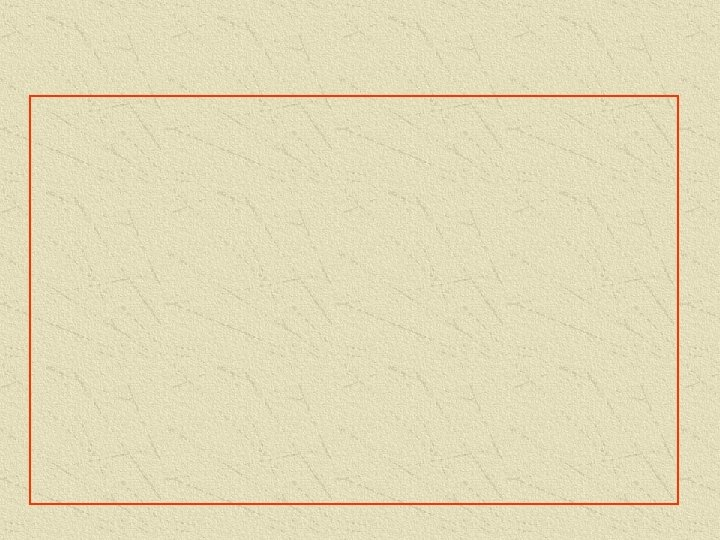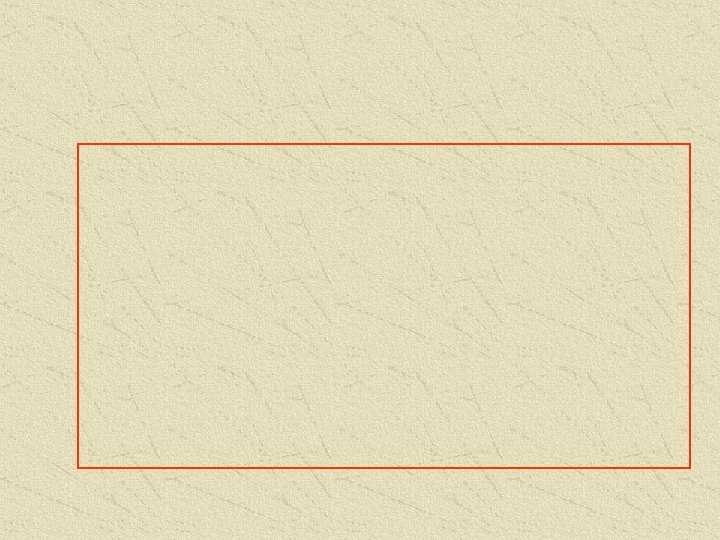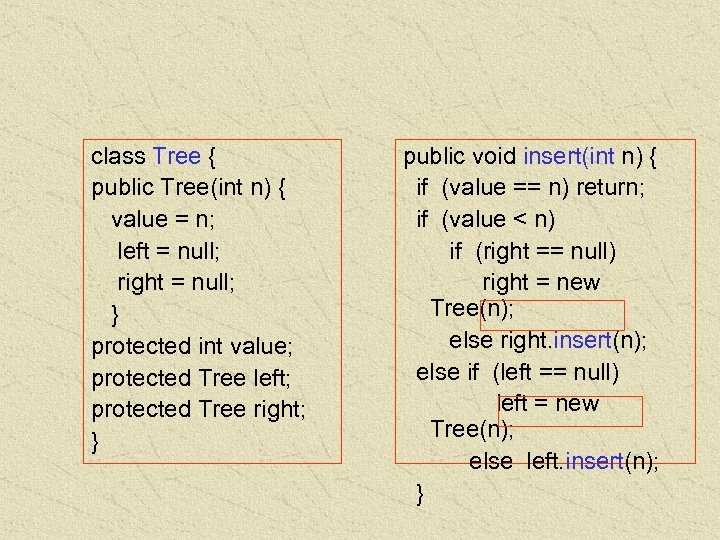class Tree { public Tree(int n) { value = n; left = null; right = null; } protected int value; protected Tree left; protected Tree right; } public void insert(int n) { if (value == n) return; if (value < n) if (right == null) right = new Tree(n); else right. insert(n); else if (left == null) left = new Tree(n); else left. insert(n); }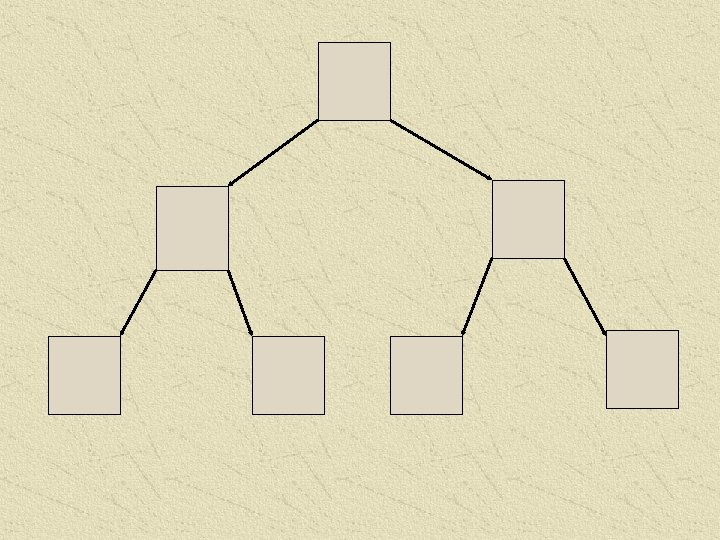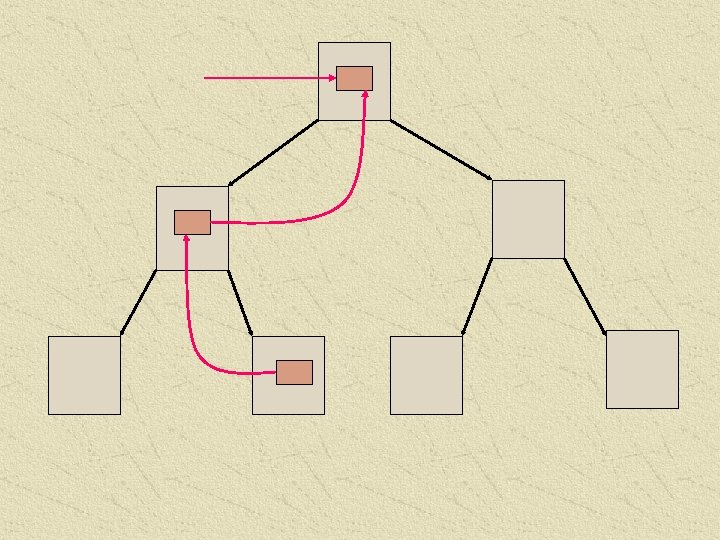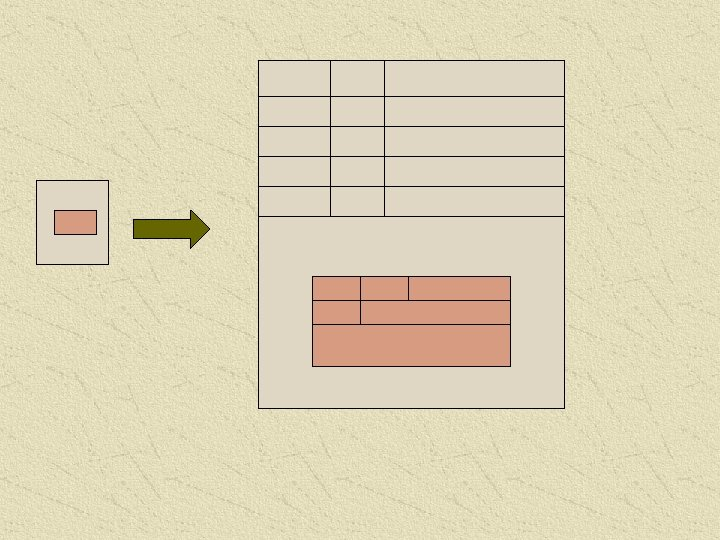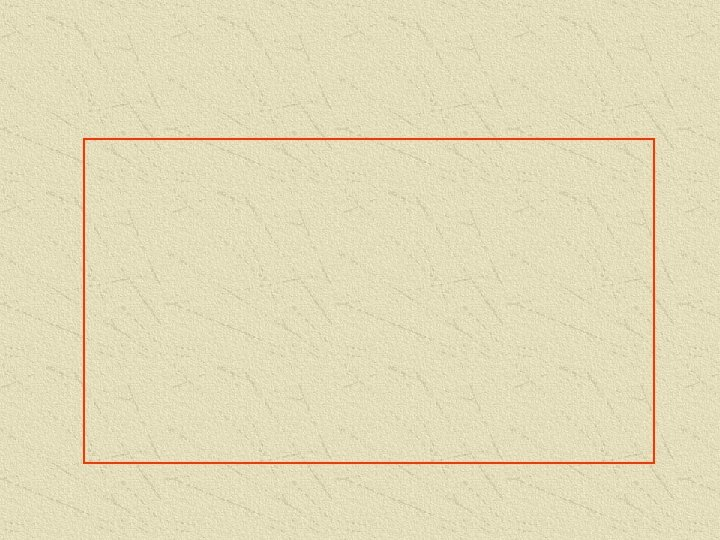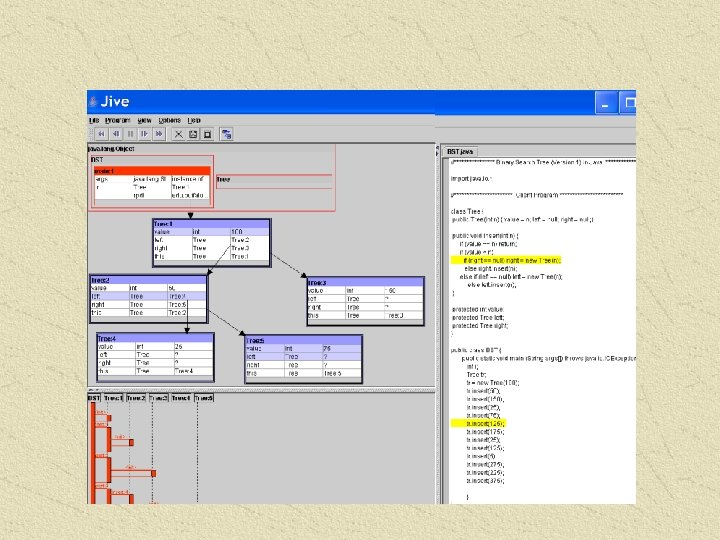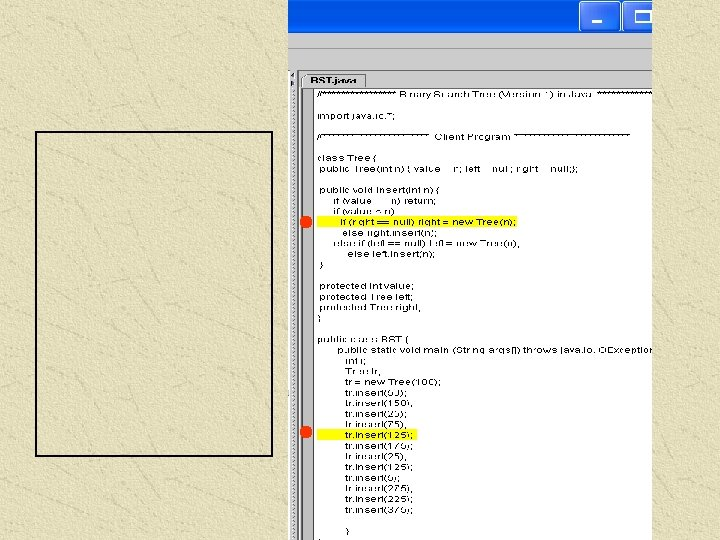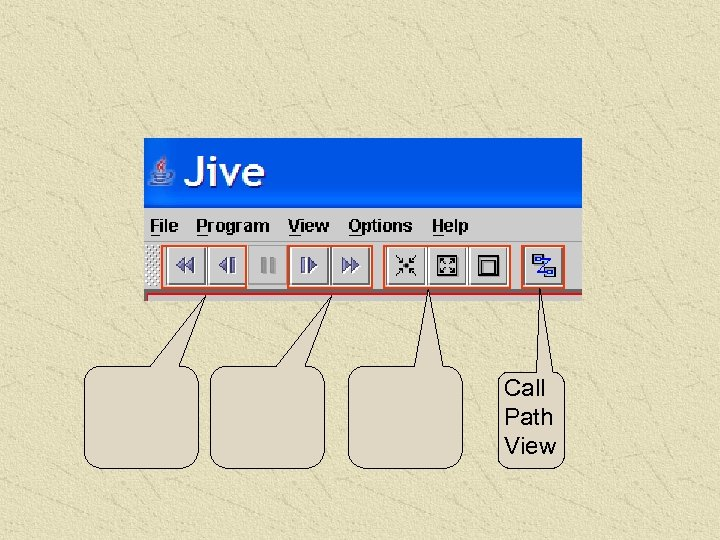Call Path View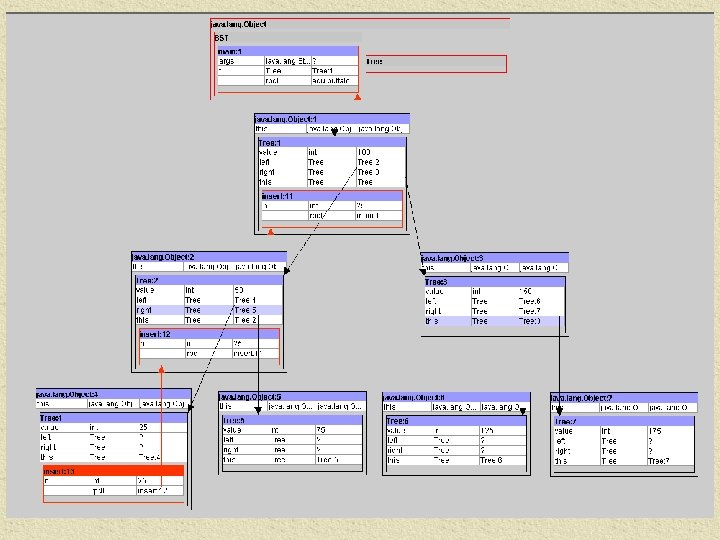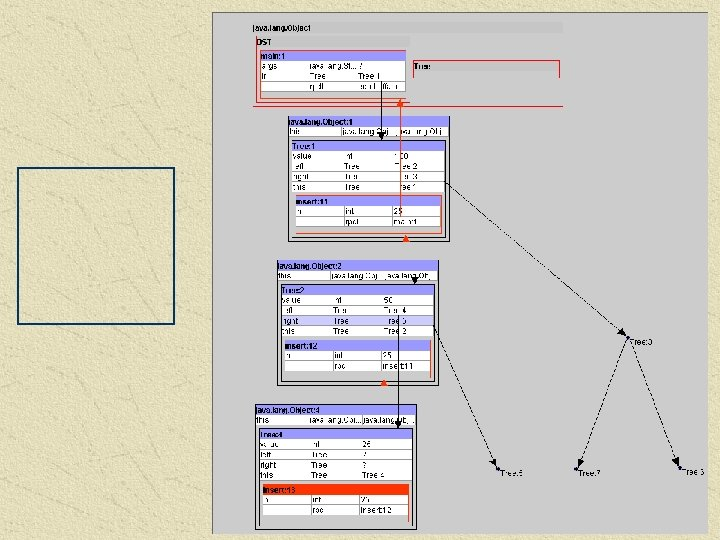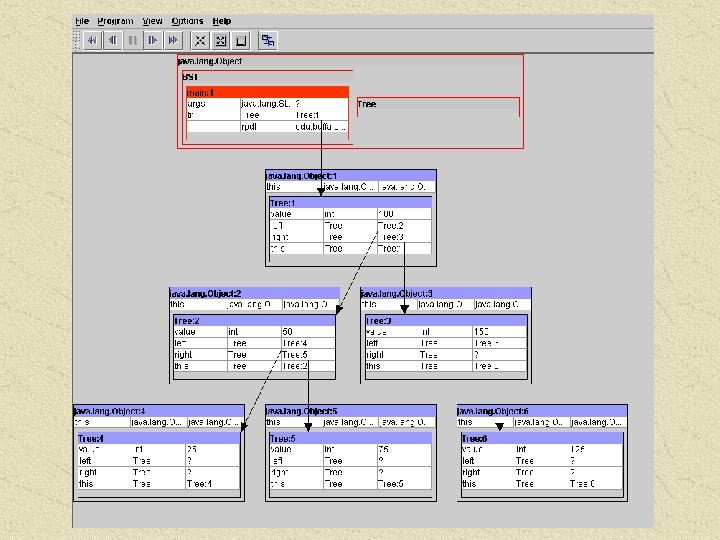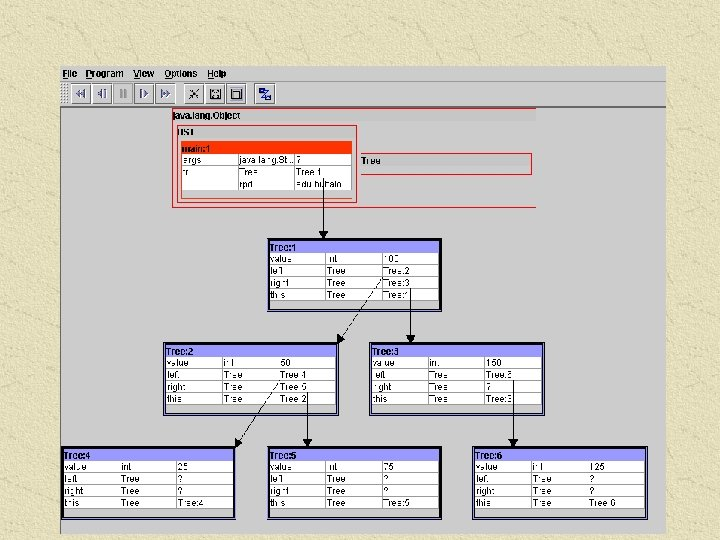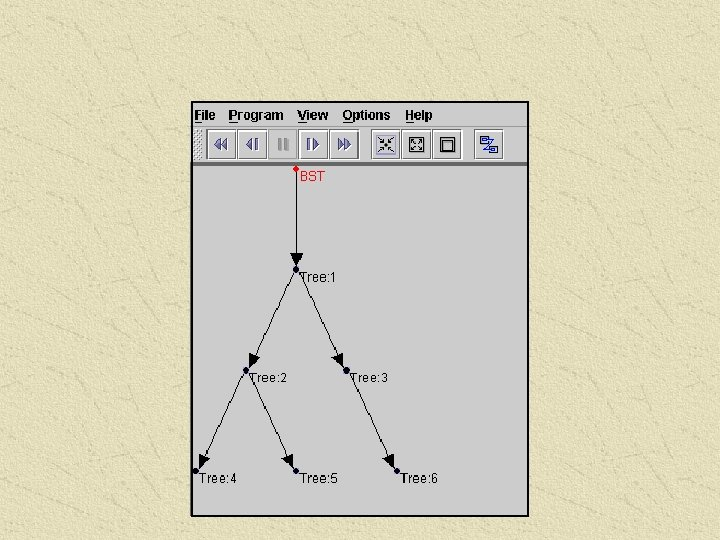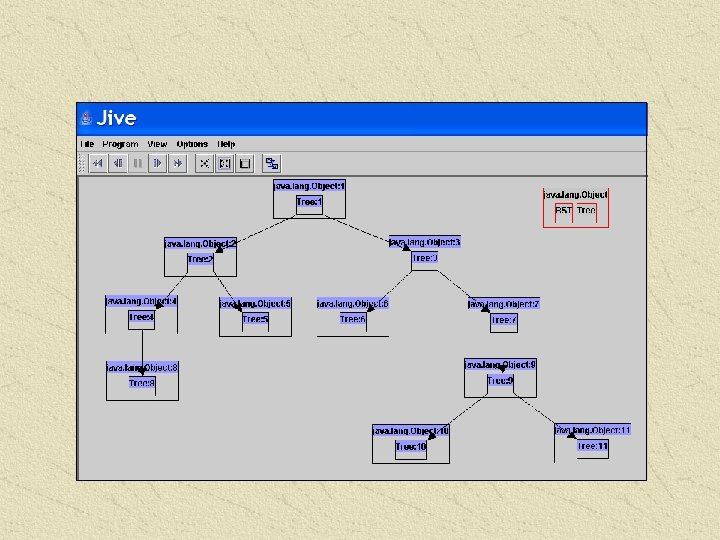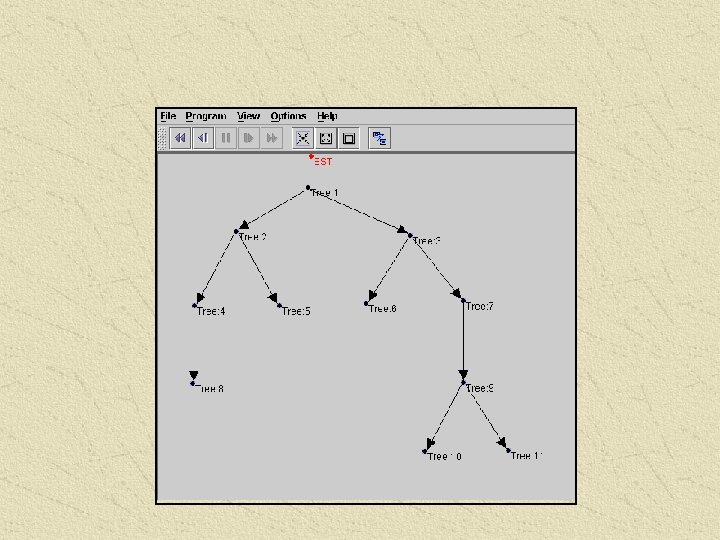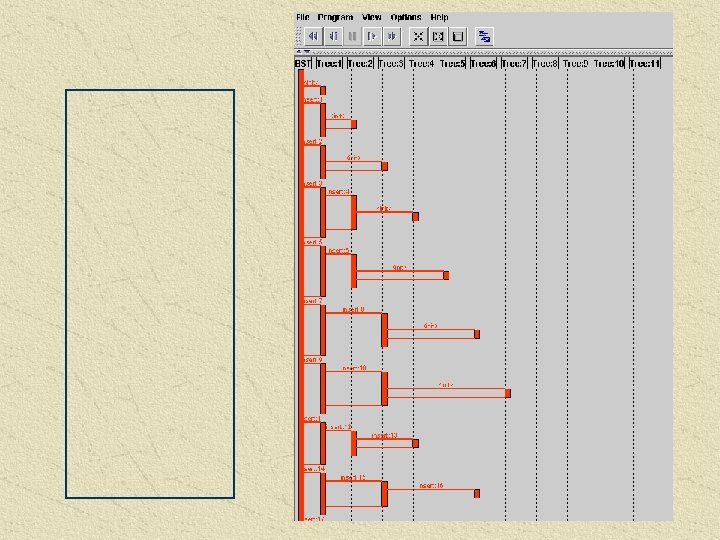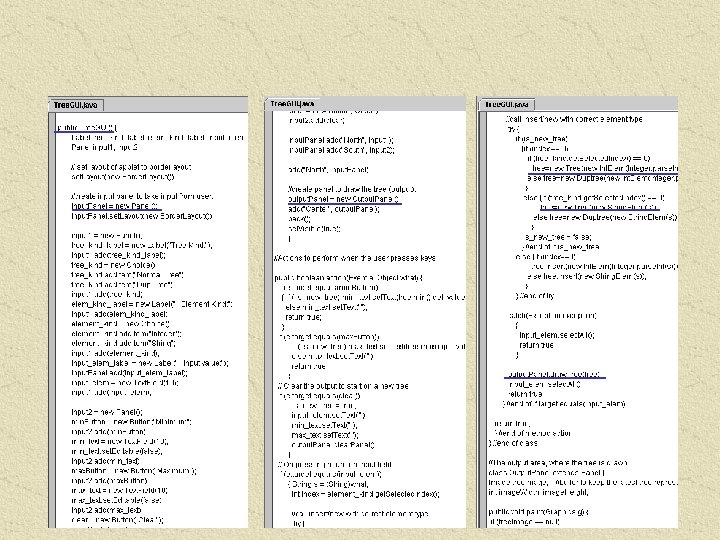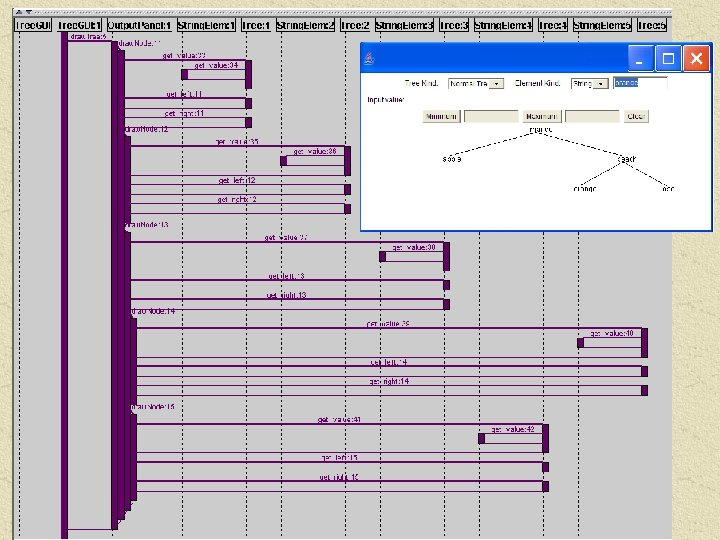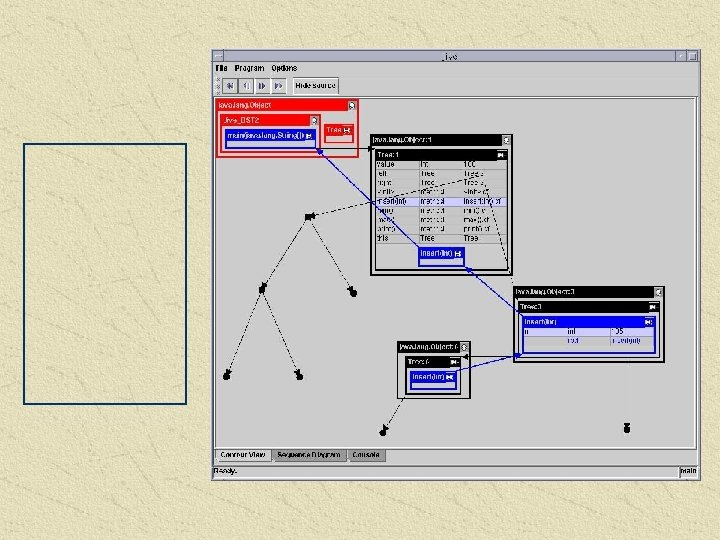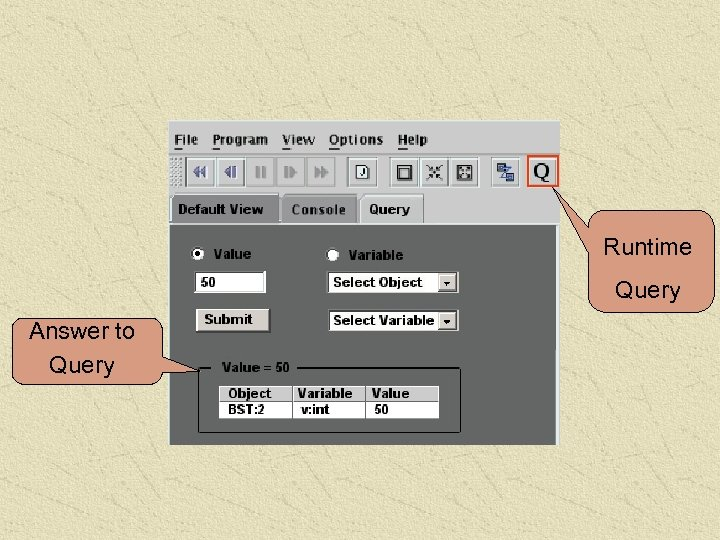Runtime Query Answer to Query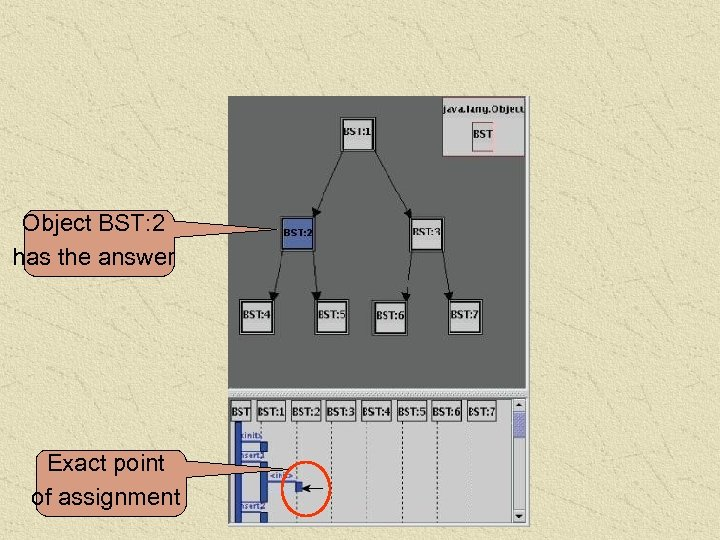Object BST: 2 has the answer Exact point of assignment